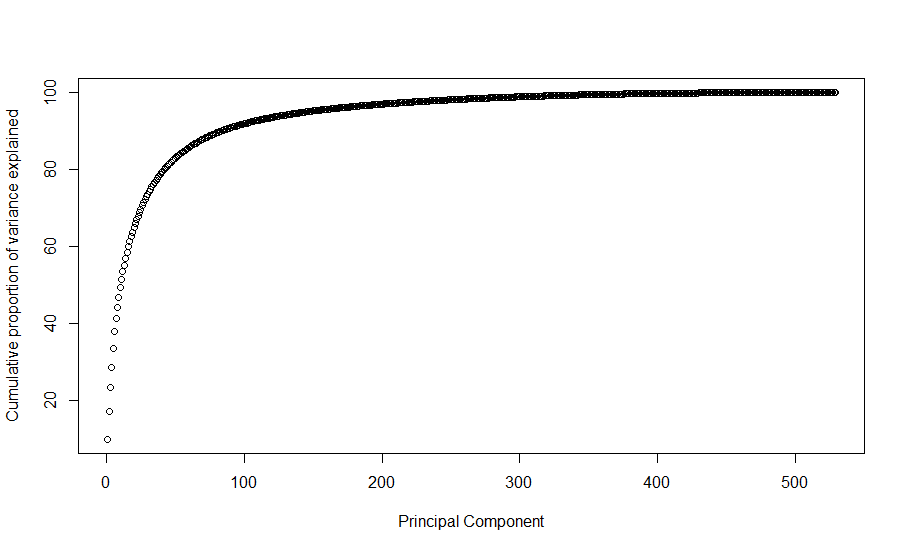## PCA实现过程梳理以及降噪处理

R语言中，PCA一般常用的基础函数是`prcomp``princomp`，两者有些差别：

• prcomp适用于R mode和Q mode，而princomp只适用于R mode
• prcomp基于奇异值分解（SVD），求最小二乘的优化解（最小化的结果要求寻找一个正交矩阵AB,记作H[q]，H[q]为投影矩阵，把每个点X[i]投影到q维结构H[q]x[i],这是由V[q]的列限定的x[i]的正交投影）
• princomp是基于相关系数矩阵的特征值分解，方差最大化方法（本质上是求原始变量协方差/相关系数矩阵的特征值和特征向量，第i主成分对应着协方差的第i大特征值，投影方向为该特征值对应的特征向量，而特征值等于相应主成分的方差，因此代表着对应主成分分解原始信息的多少）
• prcomp在参数中如果设定了`scale=T`，那么如果princomp结果想与prcomp保持一致，则princomp函数需加上`cor=T`参数（其实原因在于cor和cov之间刚好相差了一个scale）

R mode是指基于variable分析，一般来研究variable之间的关系，当数据集的observation数目大于variable时；Q mode是指基于observation分析，一般来研究observation之间的的关系，当数据集的varibale数目大于observation时

``````data(USArrests)
dim(USArrests)

res1 <- prcomp(USArrests, scale = T)
sd <- res1\$sdev
loadings <- res1\$rotation
scores <- res1\$x
``````

``````R <- cor(USArrests)

# find the eigenvalues and eigenvectors of correlation matrix
myEig <- eigen(R)

# eigenvalues stored in myEig\$values
# eigenvectors (loadings) stored in myEig\$vectors
loadingsLONG <- myEig\$vectors
rownames(loadingsLONG) <- colnames(USArrests)

# calculating singular values from eigenvalues
sdLONG <- sqrt(myEig\$values)

# transforming data to zero mean and unit variance
X <- apply(USArrests, 2, function(x){
(x - mean(x))/sd(x)
})

# calculating scores from eigenanalysis results
scoresLONG <- X %*% loadingsLONG
``````

``````range(sd - sdLONG)
range(loadings - loadingsLONG)
range(scores - scoresLONG)
``````

``````require(data.table)
mnist_data=fread('../Desktop/all/train.csv')
``````

``````mnist_data2 <- mnist_data[,-(which((1:784)%%28<=2|(1:784)%%28>=26|(1:784)%/%28<=2|(1:784)%/%28>=26)+1), with=F]
``````

``````pca_res <- prcomp(mnist_data2[,(2:ncol(mnist_data2)), with=F], center = T, scale. = F)
plot(cumsum(pca_res\$sdev^2)/sum(pca_res\$sdev^2)*100,
ylab = 'Cumulative proportion of variance explained', xlab = "Principal Component")
````````````pc_use <- 300
trunc <- pca_res\$x[,1:pc_use] %*% t(pca_res\$rotation[,1:pc_use])
trunc <- scale(trunc, center = -pca_res\$center, scale = pca_res\$scale)
dim(trunc)
res <- data.table(mnist_data2\$label, trunc)
``````

``````par(mfrow=c(3,3))
for (i in 1:9) {
##Changing i-th row to matrix
set.seed(123456)
rad <- sample(which(mnist_data[,1] == i), 1)
mat <- matrix(as.numeric(mnist_data[rad, 785:2]), nrow = 28, ncol = 28, byrow = F)
##Inverting row order
mat <- mat[nrow(mat):1,]
##plot
image(mat,main=paste0('This is a ', i))
}
````````````par(mfrow=c(3,3))
for (i in 1:9) {
set.seed(123456)
rad <- sample(which(res[,1] == i), 1)
mat <- matrix(as.numeric(res[rad, 530:2]), nrow = 23, ncol = 23, byrow = F)
mat <- mat[nrow(mat):1,]
image(mat,main=paste0('This is a ', i))
}
``````